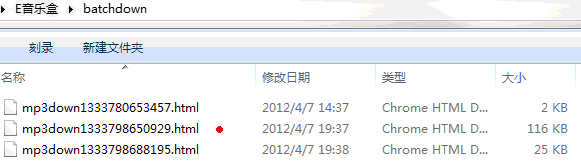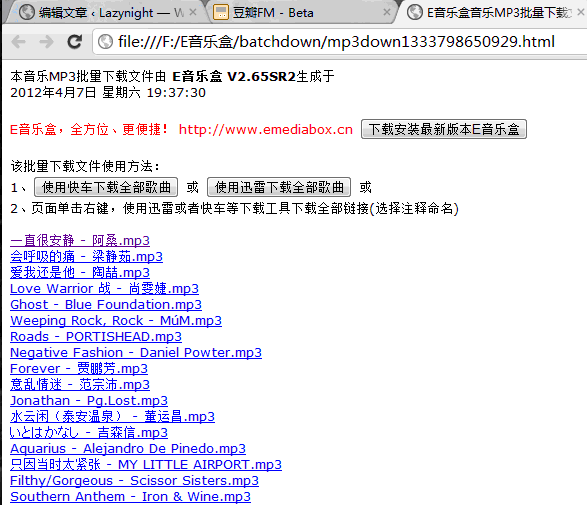# 批量下载豆瓣电台红心歌曲

1.打开Firefox，登录豆瓣电台，进入红心界面。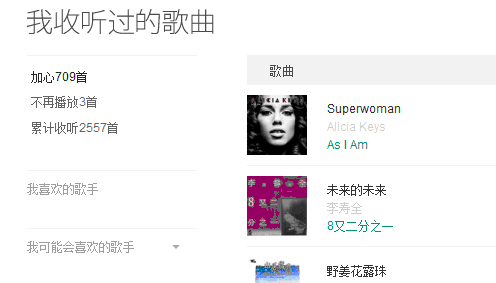2.打开暂存器，如图3.复制一下代码到暂存器中，下边的tpage=48，这个48是你的歌单总页数，请自行更改。

```var tpage = 48;
var page = 1;
var n = 0;
var songNum=0;
for(page=1;page<=tpage;page++) {
n = (page - 1) * 15;
var url = "http://douban.fm/mine?start=" + n + "&type=liked";
\$.ajax({
type:"GET"
,url:url
,async:false
,dataType:"html"
, success:function(html){
songNum+=\$(html).find("div.song_info").length;
\$(html).find("div.song_info").each(function(s) {
var d = "";
d=\$(this).find("p.song_title").first().text()+"-"+ \$(this).find("p.performer").first().text()+"<br>";
});
}
});
}```

```var tpage = 48;
var page = 1;
var n = 0;
var songNum=0;
for(page=1;page<=tpage;page++) {
n = (page - 1) * 15;
var url = "http://douban.fm/mine?start=" + n + "&type=liked";
\$.ajax({
type:"GET"
,url:url
,async:false
,dataType:"html"
, success:function(html){
songNum+=\$(html).find("div.song_info").length;
\$(html).find("div.song_info").each(function(s) {
var d = "";
d=\$(this).find("p.song_title").first().text()+"-"+
\$(this).find("p.performer").first().text()+"-"+
\$(this).find("p.source>a").first().text()+"<br>";
});
}
});
}```

Chrome下使用代码方法（不推荐使用Chrome，请求速度很慢，Firefox很快）：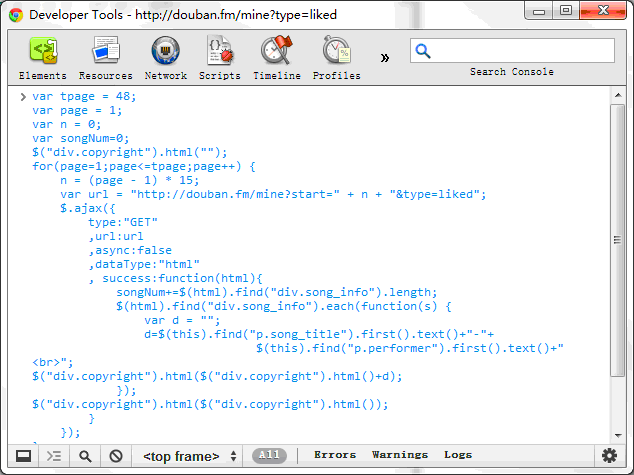4.点击暂存器工具栏的”执行”下的”运行”按钮。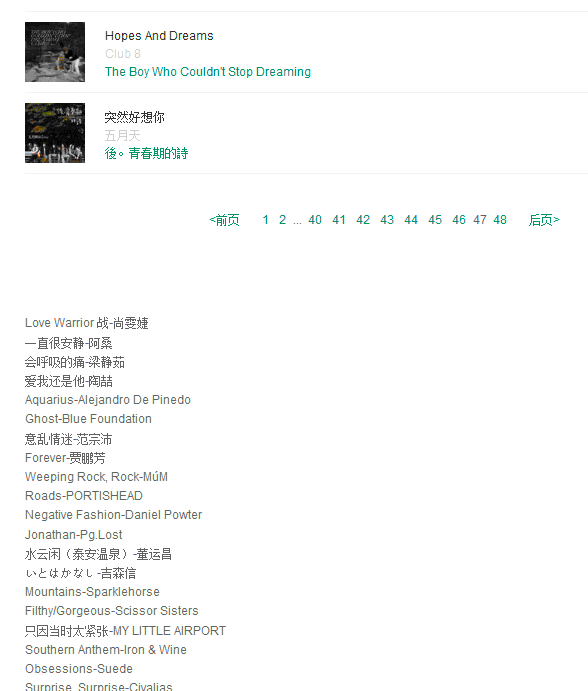5.复制歌单，保存到一个txt文件中，打开E-musicBox，导入文件列表，如图：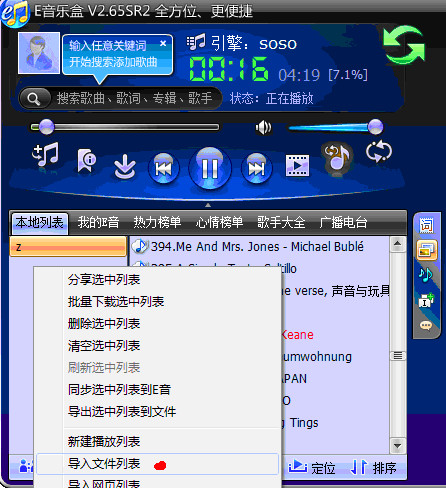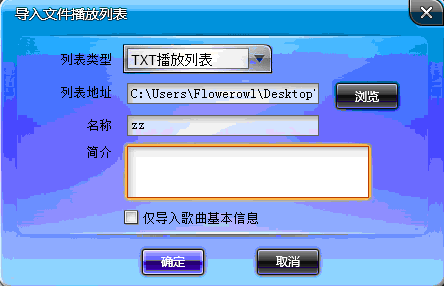6.现在导入歌单了，开始下载：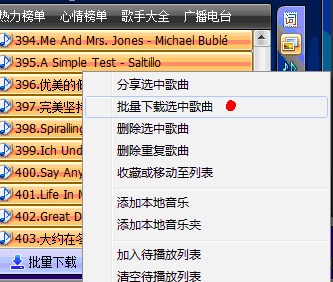7.之后会生成一个html文件，打开，用迅雷批量下载，这里我推荐使用迅雷5.83，因为之后的版本不能自动批量使用注释命名（如果你能忍受乱码命名文件很过瘾的话可以用迅雷7）。